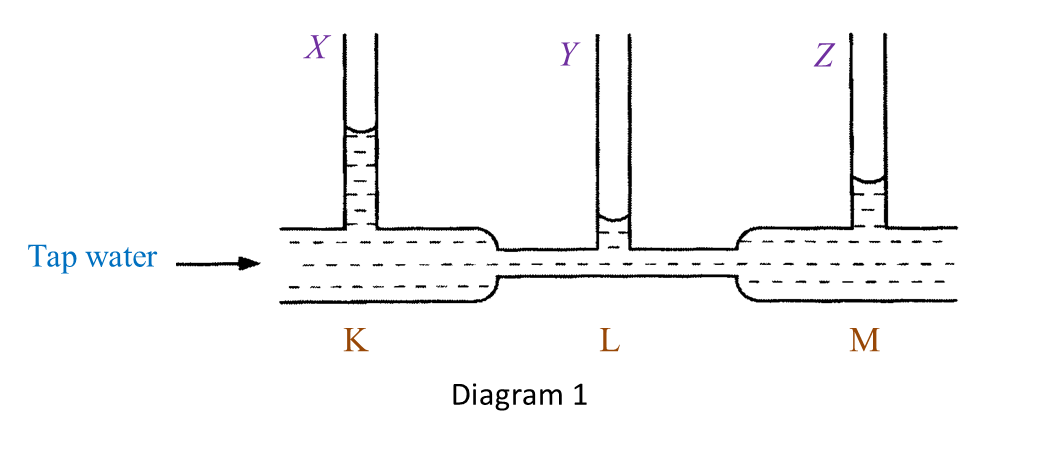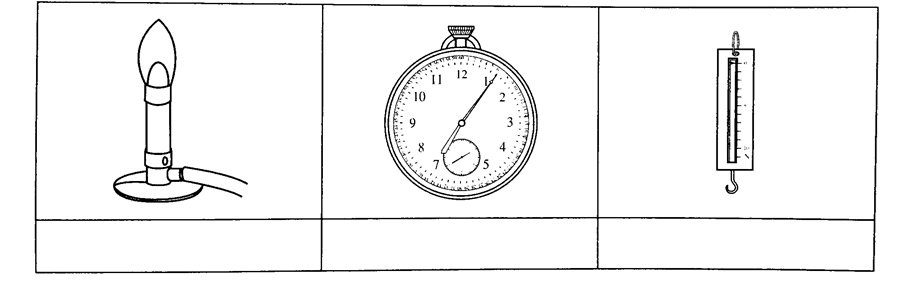# The Motion of Vehicles in the Air (Structured Questions)

Question 1:
Diagram 1 shows an experiment to study Bernoulli’s Principle.(a)(i) State one observation of the water level in Diagram 1.
(ii) State one inference that can be made based on the observation in (a)(i).
(b) State the responding variable in this experiment.
(c) Based on this experiment, state the operational definition for Bernoulli’s Principle.
(d) Mark (\/) in the boxes provided to show the apparatus where the Bernoulli’s Principle is applied.(a)(i)
– The water level in the vertical tube Y is the lowest.
– The water level in the vertical tube X is the highest.

(a)(ii)
– The water at the base of the vertical tube Y exerts the lowest pressure.
– The water at the base of the vertical tube X exerts the highest pressure.

(b)
The pressure exerted by the moving water.

(c)
Bernoulli’s Principle states that pressure in a liquid decreases when the speed of the liquid increases.

(d)